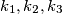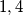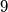### IMO Shortlist 1984 problem 18

Kvaliteta:
Avg: 0,0
Težina:
Avg: 0,0
Inside triangle$ABC$ there are three circles$k_1, k_2, k_3$ each of which is tangent to two sides of the triangle and to its incircle$k$. The radii of$k_1, k_2, k_3$ are$1, 4$, and$9$. Determine the radius of$k.$
Izvor: Međunarodna matematička olimpijada, shortlist 1984